# Multidimensional Arrays Multidimensional array is the array with

• Slides: 27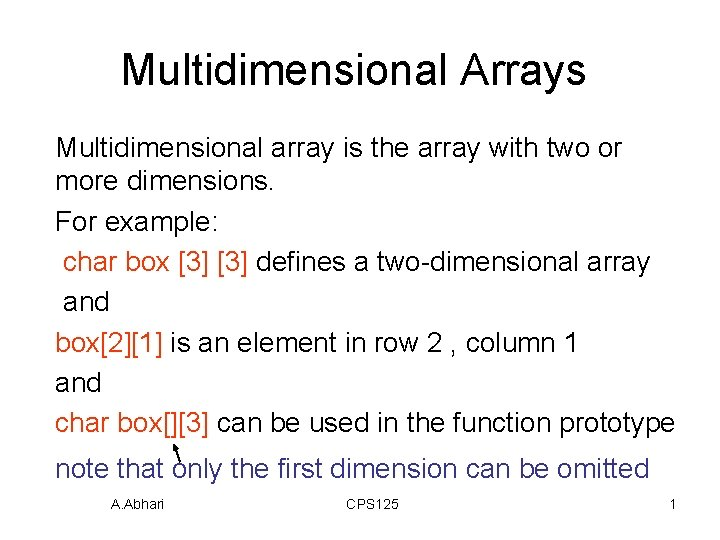Multidimensional Arrays Multidimensional array is the array with two or more dimensions. For example: char box  defines a two-dimensional array and box is an element in row 2 , column 1 and char box[] can be used in the function prototype note that only the first dimension can be omitted A. Abhari CPS 125 1Multidimensional Arrays • For example : double table [NROWS][NCOLS]; Can be used as parameter in the function prototype as: void process_martrix( int in[ ], int out[ ], int nrows) A. Abhari CPS 125 2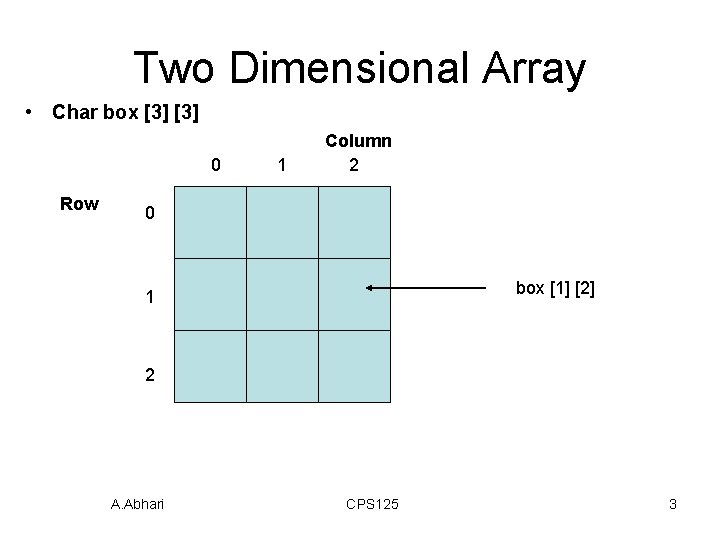Two Dimensional Array • Char box  0 Row 1 Column 2 0 box   1 2 A. Abhari CPS 125 3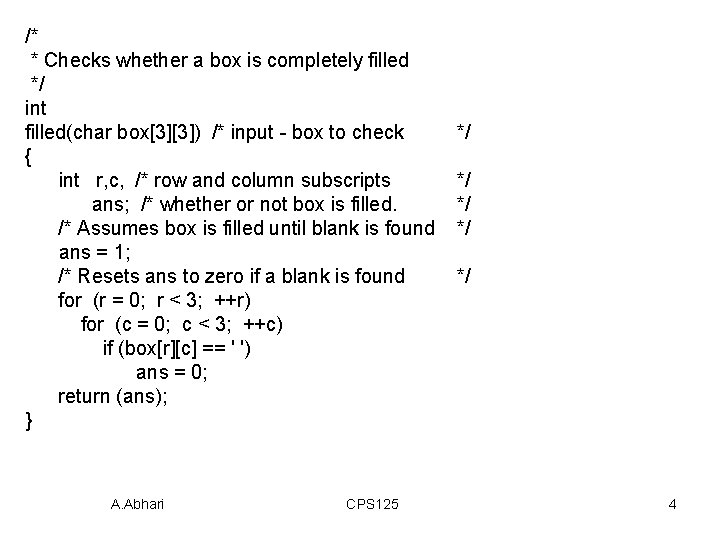/* * Checks whether a box is completely filled */ int filled(char box) /* input - box to check { int r, c, /* row and column subscripts ans; /* whether or not box is filled. /* Assumes box is filled until blank is found ans = 1; /* Resets ans to zero if a blank is found for (r = 0; r < 3; ++r) for (c = 0; c < 3; ++c) if (box[r][c] == ' ') ans = 0; return (ans); } A. Abhari CPS 125 */ */ */ 4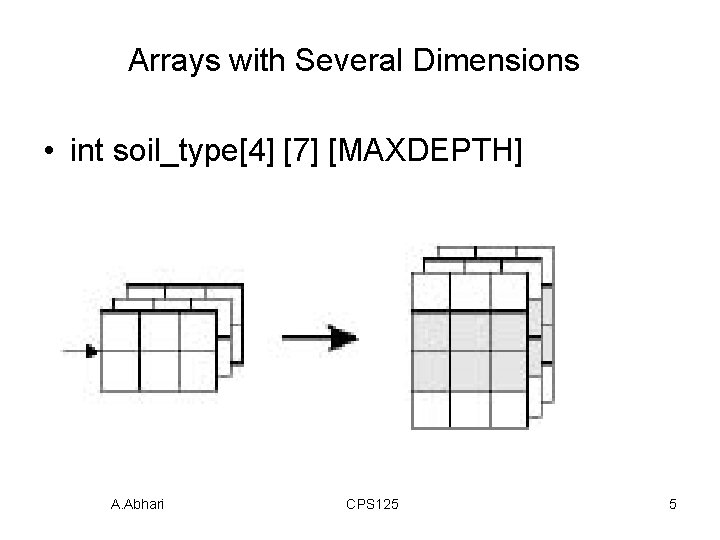Arrays with Several Dimensions • int soil_type  [MAXDEPTH] A. Abhari CPS 125 5Case Study: Cellular Telephone System • Problem: Finding the best way to build a cellular network. There is some marketing data that predicts the demand will be at tree time of interest. There are only 10 transmitters and there is a need for a program to help analyzing call demand data. A. Abhari CPS 125 6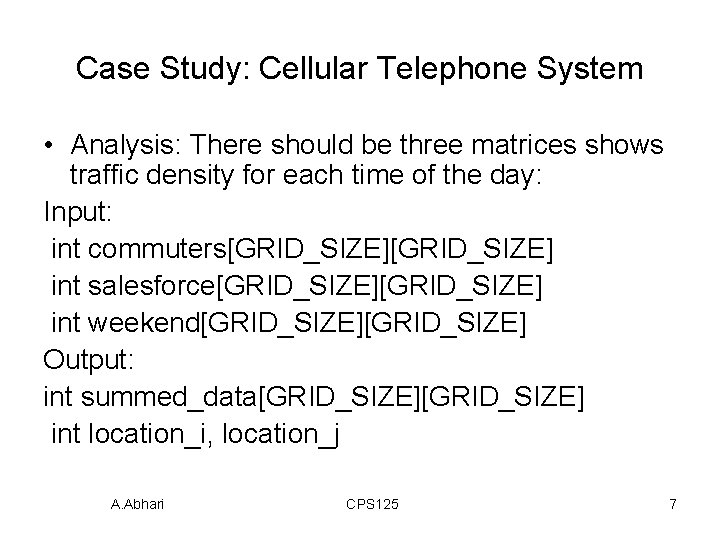Case Study: Cellular Telephone System • Analysis: There should be three matrices shows traffic density for each time of the day: Input: int commuters[GRID_SIZE] int salesforce[GRID_SIZE] int weekend[GRID_SIZE] Output: int summed_data[GRID_SIZE] int location_i, location_j A. Abhari CPS 125 7Case Study: Cellular Telephone System • 1. 2. 3. Design: initial algorithm: Get traffic data for three time period Get the weights from user Multiply weight by each matrix entry and store the sum in the summed data 4. Find highest valued cells in the summed data and display them as the pair of location_i and location_j • Implementation A. Abhari CPS 125 8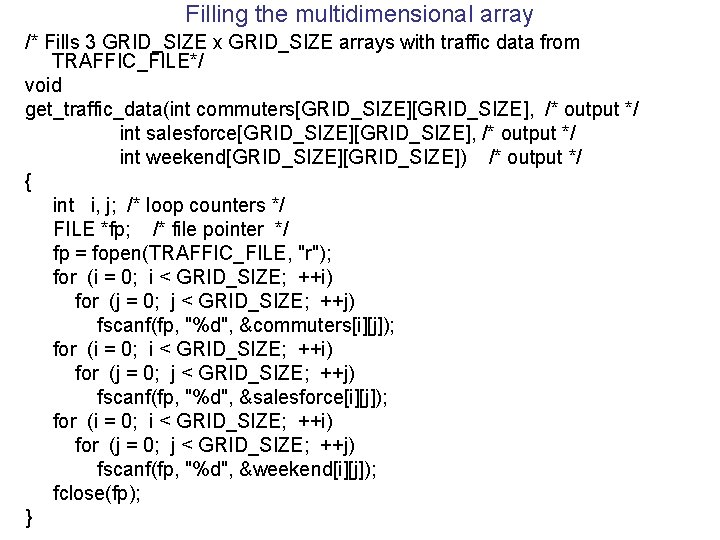Filling the multidimensional array /* Fills 3 GRID_SIZE x GRID_SIZE arrays with traffic data from TRAFFIC_FILE*/ void get_traffic_data(int commuters[GRID_SIZE], /* output */ int salesforce[GRID_SIZE], /* output */ int weekend[GRID_SIZE]) /* output */ { int i, j; /* loop counters */ FILE *fp; /* file pointer */ fp = fopen(TRAFFIC_FILE, "r"); for (i = 0; i < GRID_SIZE; ++i) for (j = 0; j < GRID_SIZE; ++j) fscanf(fp, "%d", &commuters[i][j]); for (i = 0; i < GRID_SIZE; ++i) for (j = 0; j < GRID_SIZE; ++j) fscanf(fp, "%d", &salesforce[i][j]); for (i = 0; i < GRID_SIZE; ++i) for (j = 0; j < GRID_SIZE; ++j) fscanf(fp, "%d", &weekend[i][j]); fclose(fp); }Modifying the multidimensional array /* Computes and displays the weighted, summed_data */ for (i = 0; i < GRID_SIZE; ++i) for (j = 0; j < GRID_SIZE; ++j) summed_data[i][j] = commuter_weight* commuters[i][j] + salesforce_weight * salesforce[i][j] + weekend_weight * weekend[i][j]; A. Abhari CPS 125 10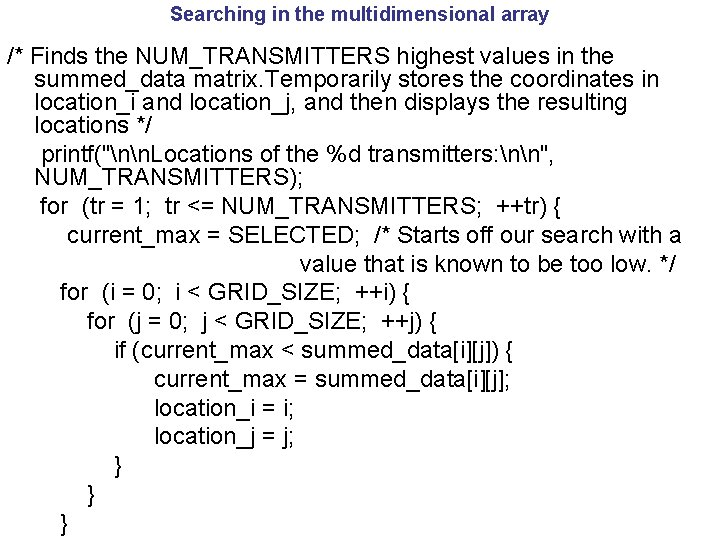Searching in the multidimensional array /* Finds the NUM_TRANSMITTERS highest values in the summed_data matrix. Temporarily stores the coordinates in location_i and location_j, and then displays the resulting locations */ printf("nn. Locations of the %d transmitters: nn", NUM_TRANSMITTERS); for (tr = 1; tr <= NUM_TRANSMITTERS; ++tr) { current_max = SELECTED; /* Starts off our search with a value that is known to be too low. */ for (i = 0; i < GRID_SIZE; ++i) { for (j = 0; j < GRID_SIZE; ++j) { if (current_max < summed_data[i][j]) { current_max = summed_data[i][j]; location_i = i; location_j = j; } } }Printing the contents of multidimensional array /* * Displays contents of a GRID_SIZE x GRID_SIZE matrix of integers */ void print_matrix(int matrix[GRID_SIZE]) { int i, j; /* loop counters */ for (i = 0; i < GRID_SIZE; ++i) { for (j = 0; j < GRID_SIZE; ++j) printf("%3 d ", matrix[i][j]); printf("n"); } } A. Abhari CPS 125 12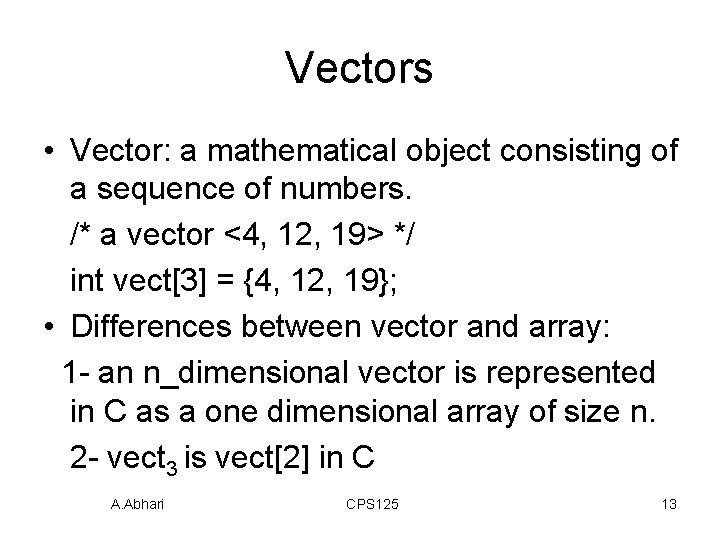Vectors • Vector: a mathematical object consisting of a sequence of numbers. /* a vector <4, 12, 19> */ int vect = {4, 12, 19}; • Differences between vector and array: 1 - an n_dimensional vector is represented in C as a one dimensional array of size n. 2 - vect 3 is vect in C A. Abhari CPS 125 13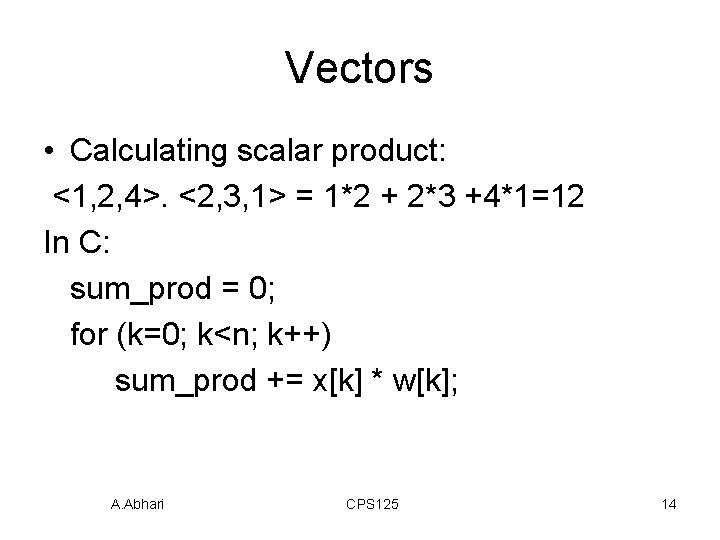Vectors • Calculating scalar product: <1, 2, 4>. <2, 3, 1> = 1*2 + 2*3 +4*1=12 In C: sum_prod = 0; for (k=0; k<n; k++) sum_prod += x[k] * w[k]; A. Abhari CPS 125 14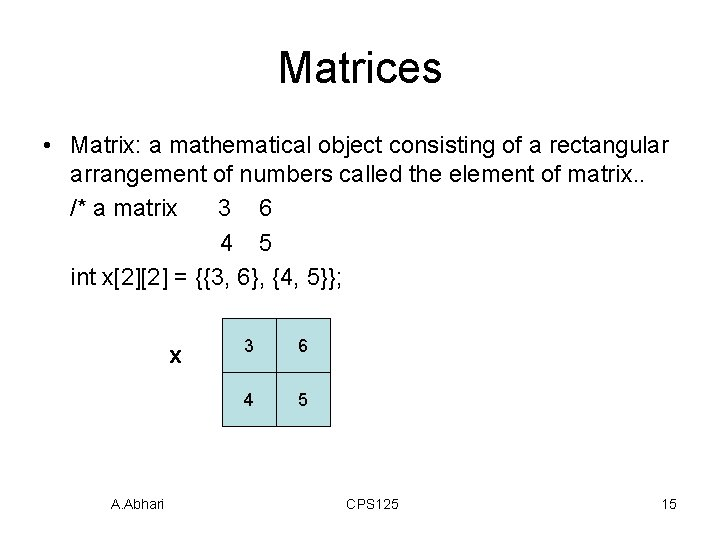Matrices • Matrix: a mathematical object consisting of a rectangular arrangement of numbers called the element of matrix. . /* a matrix 3 6 4 5 int x = {{3, 6}, {4, 5}}; x A. Abhari 3 6 4 5 CPS 125 15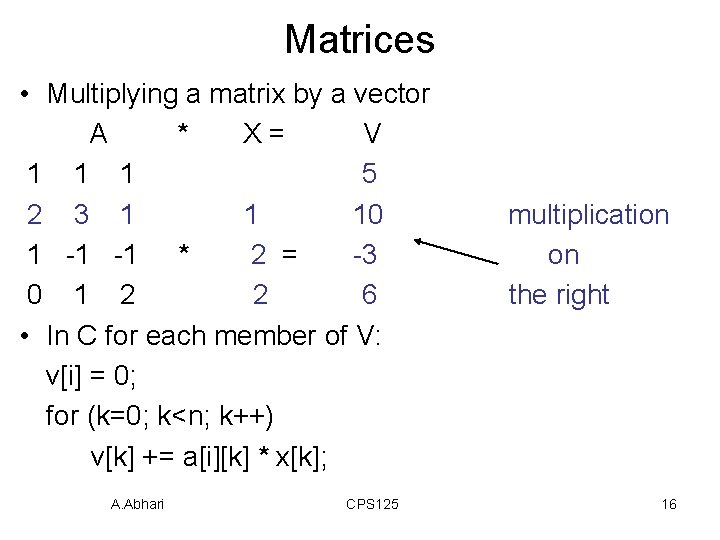Matrices • Multiplying a matrix by a vector A * X= V 1 1 1 5 2 3 1 1 10 1 -1 -1 * 2 = -3 0 1 2 2 6 • In C for each member of V: v[i] = 0; for (k=0; k<n; k++) v[k] += a[i][k] * x[k]; A. Abhari CPS 125 multiplication on the right 16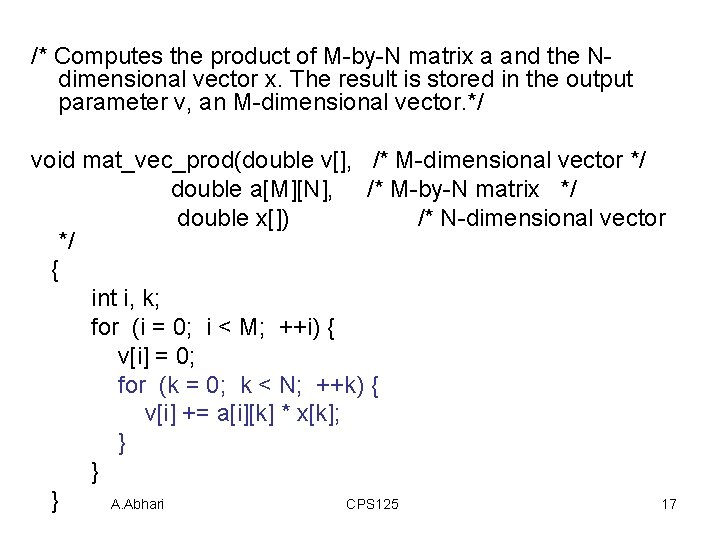/* Computes the product of M-by-N matrix a and the Ndimensional vector x. The result is stored in the output parameter v, an M-dimensional vector. */ void mat_vec_prod(double v[], /* M-dimensional vector */ double a[M][N], /* M-by-N matrix */ double x[]) /* N-dimensional vector */ { int i, k; for (i = 0; i < M; ++i) { v[i] = 0; for (k = 0; k < N; ++k) { v[i] += a[i][k] * x[k]; } } } A. Abhari CPS 125 17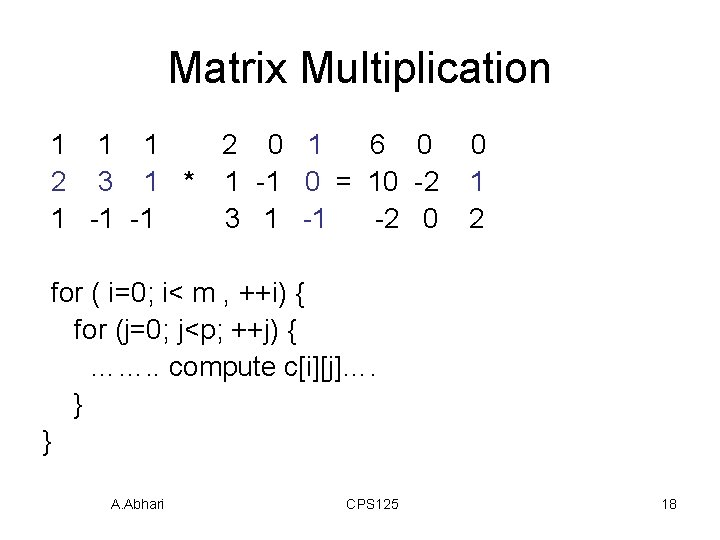Matrix Multiplication 1 1 1 2 0 1 6 0 2 3 1 * 1 -1 0 = 10 -2 1 -1 -1 3 1 -1 -2 0 0 1 2 for ( i=0; i< m , ++i) { for (j=0; j<p; ++j) { ……. . compute c[i][j]…. } } A. Abhari CPS 125 18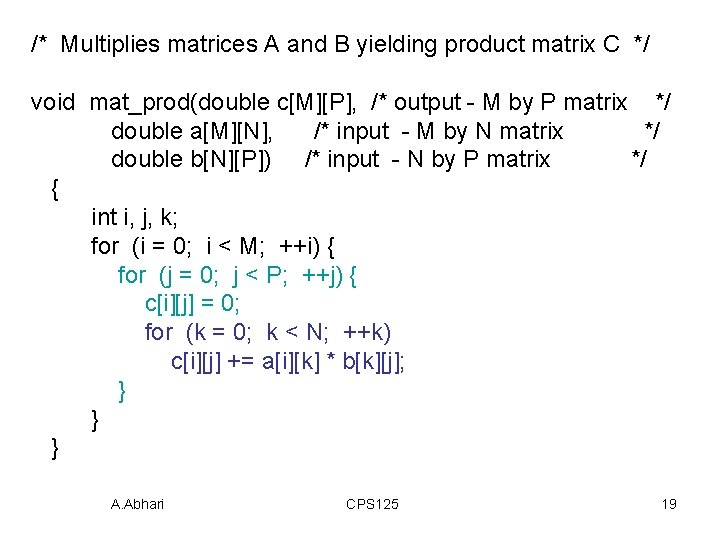/* Multiplies matrices A and B yielding product matrix C */ void mat_prod(double c[M][P], /* output - M by P matrix */ double a[M][N], /* input - M by N matrix */ double b[N][P]) /* input - N by P matrix */ { int i, j, k; for (i = 0; i < M; ++i) { for (j = 0; j < P; ++j) { c[i][j] = 0; for (k = 0; k < N; ++k) c[i][j] += a[i][k] * b[k][j]; } } } A. Abhari CPS 125 19Solving System of Linear Equations • To solve many problems such as force equation in three-dimensional system we need to solve a three linear equations: A X = Y 1 1 1 x 1 4 2 3 1 * x 2 = 9 1 -1 -1 x 3 -2 It is multiplication of a matrix by a vector on the right A. Abhari CPS 125 20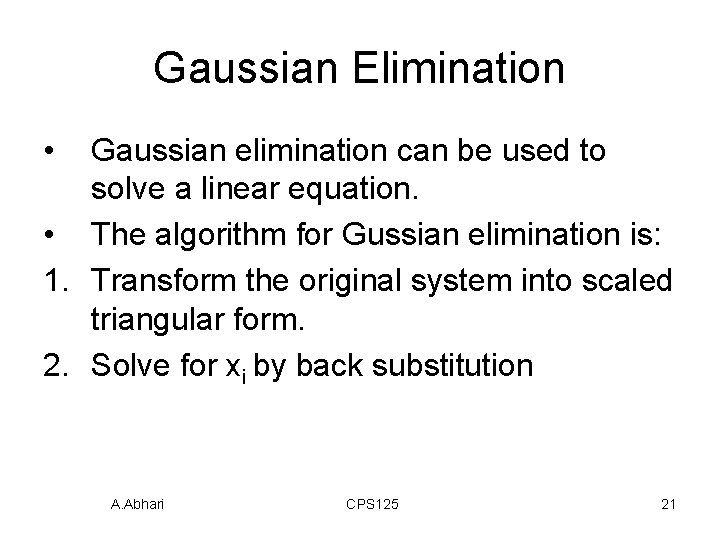Gaussian Elimination • Gaussian elimination can be used to solve a linear equation. • The algorithm for Gussian elimination is: 1. Transform the original system into scaled triangular form. 2. Solve for xi by back substitution A. Abhari CPS 125 21Gaussian Elimination triangular form 1 1 1 x 1 0 1 -1 * x 2 0 0 1 x 3 back substitution x 1 + x 2 + x 3 = 4 x 2 - x 3 = 1 A. Abhari CPS 125 = 4 1 1 22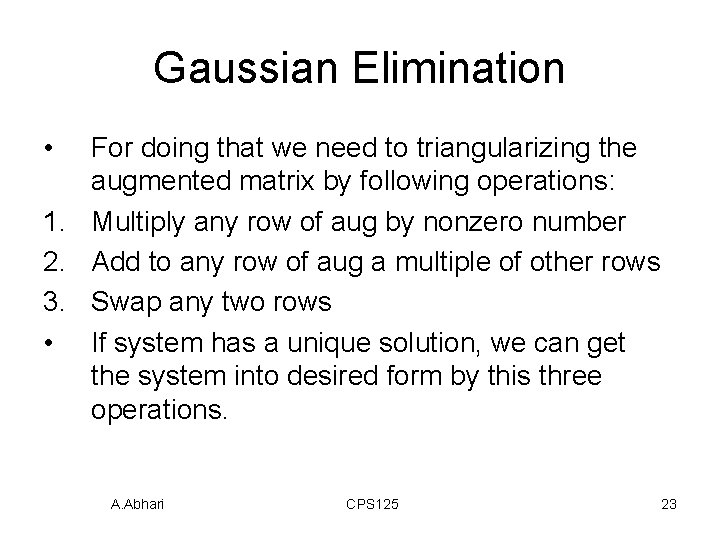Gaussian Elimination • For doing that we need to triangularizing the augmented matrix by following operations: 1. Multiply any row of aug by nonzero number 2. Add to any row of aug a multiple of other rows 3. Swap any two rows • If system has a unique solution, we can get the system into desired form by this three operations. A. Abhari CPS 125 23/* * Performs pivoting with respect to the pth row and the pth column * If no nonzero pivot can be found, FALSE is sent back through piv_foundp */ void pivot(double aug[N][N+1], /* input/output - augmented matrix */ int p, /* input - current row */ int *piv_foundp) /* output - whether or not nonzero pivot found */ { double xmax, xtemp; int j, k, max_row; /* Finds maximum pivot */ xmax = fabs(aug[p][p]); max_row = p; for (j = p+1; j < N; ++j) { if (fabs(aug[j][p]) > xmax) { xmax = fabs(aug[j][p]); max_row = j; } A. Abhari } CPS 125 24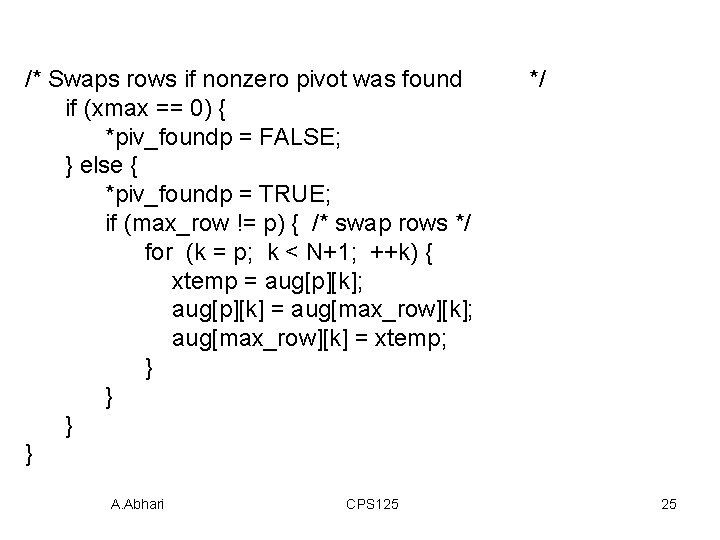/* Swaps rows if nonzero pivot was found if (xmax == 0) { *piv_foundp = FALSE; } else { *piv_foundp = TRUE; if (max_row != p) { /* swap rows */ for (k = p; k < N+1; ++k) { xtemp = aug[p][k]; aug[p][k] = aug[max_row][k]; aug[max_row][k] = xtemp; } } A. Abhari CPS 125 */ 25/* * Performs back substitution to compute a solution vector to a system of * linear equations represented by the augmented matrix aug. Assumes that * the coefficient portion of the augmented matrix has been triangularized, * and its diagonal values are all 1. */ void back_sub(double aug[N][N+1], /* input - scaled, triangularized augmented matrix */ double x[N]) /* output - solution vector */ { double sum; int i, j; x[N - 1] = aug[N - 1][N]; for (i = N - 2; i >= 0; --i) { sum = 0; for (j = i + 1; j < N; ++j) sum += aug[i][j] * x[j]; x[i] = aug[i][N] - sum; } }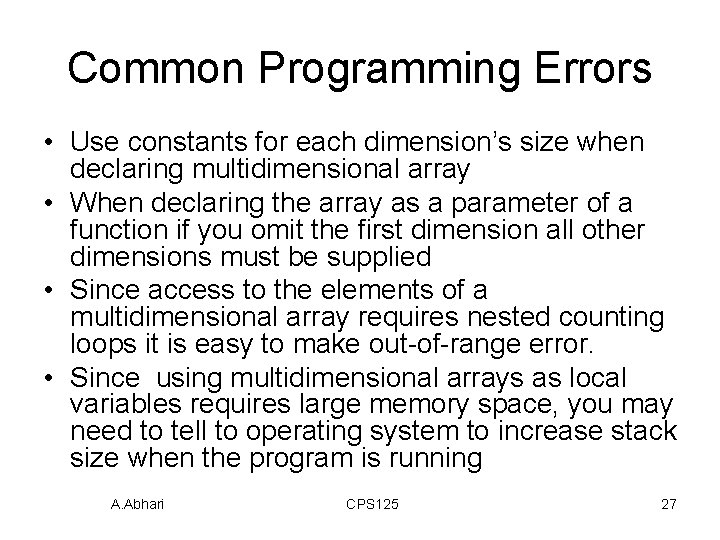Common Programming Errors • Use constants for each dimension’s size when declaring multidimensional array • When declaring the array as a parameter of a function if you omit the first dimension all other dimensions must be supplied • Since access to the elements of a multidimensional array requires nested counting loops it is easy to make out-of-range error. • Since using multidimensional arrays as local variables requires large memory space, you may need to tell to operating system to increase stack size when the program is running A. Abhari CPS 125 27